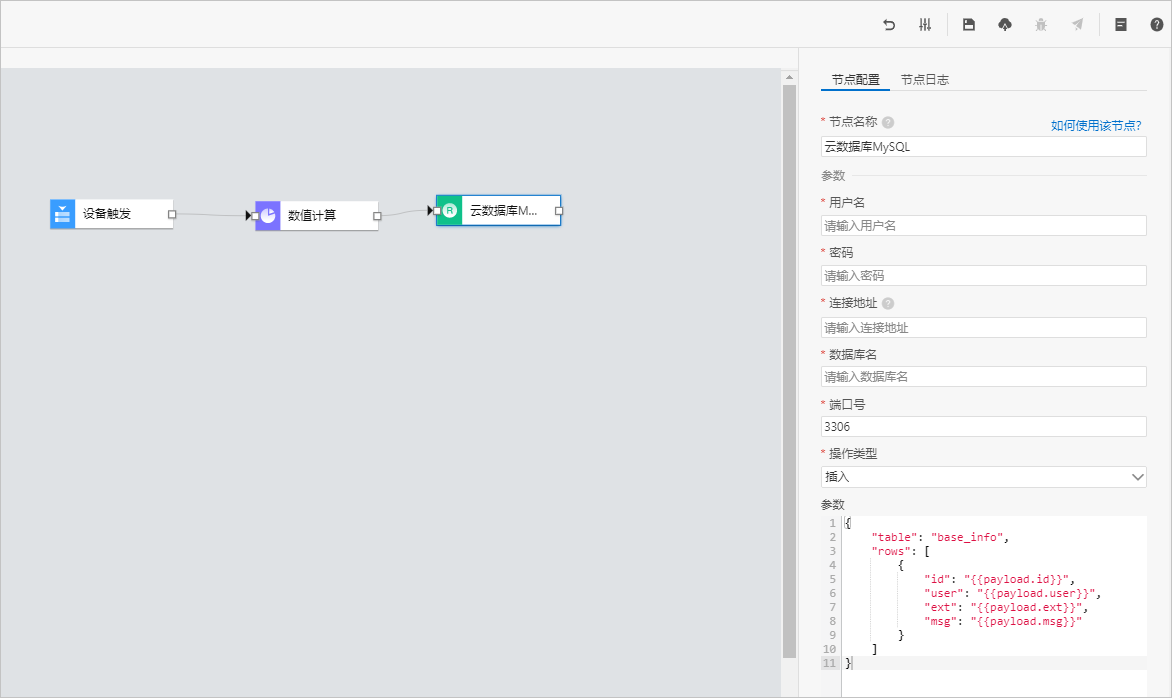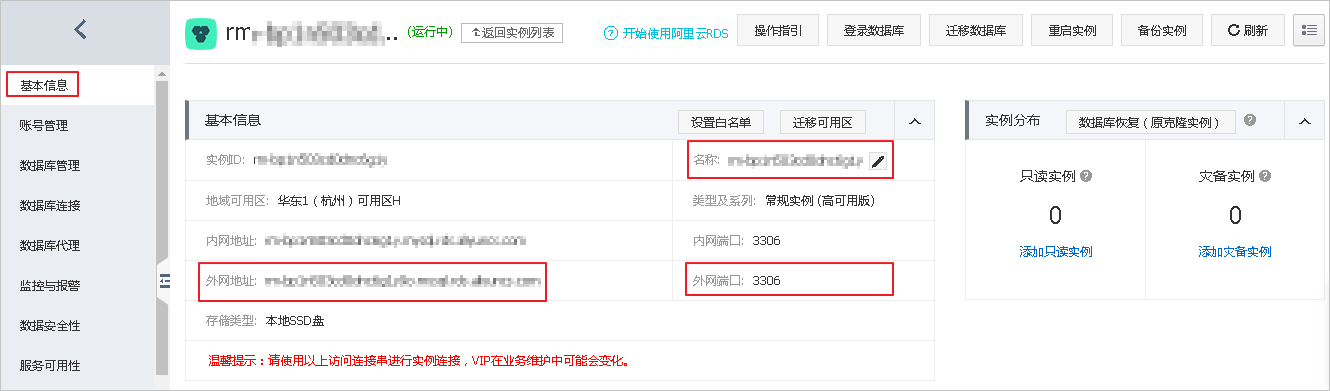## 节点配置• 插入：在数据库中插入数据。
• 查询：查询数据库中的数据。
• 删除：删除数据库中的数据。
• 更新：更新数据库中的数据。## 参数

• payload指来自上个节点的输出数据。支持使用`payload.payload对象中的某个key`来访问指定key的数据。
• query指来自输入节点的数据。例如，HTTP请求节点的请求参数，设备触发节点的设备数据。支持使用`query.参数名`来访问指定数据。
• `node.节点ID`指来自指定节点的输出数据。支持使用`node.节点ID.节点输出对象中的某个key`来访问指定key的数据。

• 插入
``````{
"table": "",  //数据表名称
"rows": [{}]  //插入的数据内容
}``````

示例

``````{
"table": "base_info",
"rows": [
{
}
]
}``````
• 查询
``````{
"table": "",        //数据表名称
"condition": {      //查询条件
"where": {},    //查询位置
"columns": [],  //查询内容
"orders": []    //排序
}
}``````

示例

``````{
"table": "base_info",
"condition": {
"where": {"id": "{{query.id}}"},
"columns": ["id", "user", "ext"],
"orders": [["id", "desc"]]
}
}
}``````
• 删除
``````{
"table": "",      //数据表名称
"condition": {    //删除条件
}
}``````

示例

``````{
"table": "base_info",
"condition": {
"id": "{{node.node_781d4a40.id}}"
}
}``````
• 更新
``````{
"table": "",          //数据表名称
"row": {},            //更新的键值对
"condition": {        //更新条件
"where": {},      //更新位置
"columns": []     //更新内容
}
}``````

示例

``````{
"table": "base_info",
"row": {"user": "xxx", "ext": "yyy"},
"condition": {
"where": {"id": "{{query.id}}"},
"columns": ["user", "ext"]
}
}
}``````